# Priors Are Useless

## NOTE.

This post contains Latex. Please install Tex the World for Chromium or other similar Tex typesetting extensions to view this post properly.

# Priors are Useless.

Priors are irrelevant. Given two different prior probabilities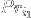, and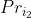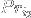for some hypothesis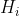.
Let their respective posterior probabilities be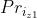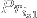and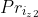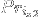.
After sufficient number of experiments, the posterior probability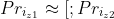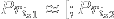.
Or More formally: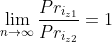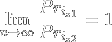.
Where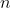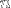is the number of experiments.
Therefore, priors are useless.
The above is true, because as we carry out subsequent experiments, the posterior probability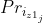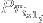gets closer and closer to the true probability of the hypothesis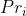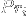. The same holds true for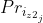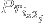. As such, if you have access to a sufficient number of experiments the initial prior hypothesis you assigned the experiment is irrelevant.

To demonstrate.
http://​​i.prntscr.com/​​hj56iDxlQSW2x9Jpt4Sxhg.png
This is the graph of the above table:
http://​​i.prntscr.com/​​pcXHKqDAS\_C2aInqzqblnA.png

In the example above, the true probability of Hypothesis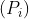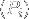is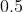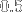and as we see, after sufficient number of trials, the differents get closer to.

To generalize from my above argument:

If you have enough information, your initial beliefs are irrelevant—you will arrive at the same final beliefs.

Because I can’t resist, a corollary to Aumann’s agreement theorem.
Given sufficient information, two rationalists will always arrive at the same final beliefs irrespective of their initial beliefs.

The above can be generalized to what I call the “Universal Agreement Theorem”:

Given sufficient evidence, all rationalists will arrive at the same set of beliefs regarding a phenomenon irrespective of their initial set of beliefs regarding said phenomenon.

Prove.

• This is totally backwards. I would phrase it, “Priors get out of the way once you have enough data.” That’s a good thing, that makes them useful, not useless. Its purpose is right there in the name—it’s your starting point. The evidence takes you on a journey, and you asymptotically approach your goal.

If priors were capable of skewing the conclusion after an unlimited amount of evidence, that would make them permanent, not simply a starting-point. That would be writing the bottom line first. That would be broken reasoning.

• “A ladder you throw away once you have climbed up it”.

• Where’s that from?

• But what exactly constitutes “enough data”? With any finite amount of data, couldn’t it be cancelled out if your prior probability is small enough?

• Yes, but that’s not the way the problem goes. You don’t fix your prior in response to the evidence in order to force the conclusion (if you’re doing it anything like right). So different people with different priors will have different amounts of evidence required: 1 bit of evidence for every bit of prior odds against, to bring it up to even odds, and then a few more to reach it as a (tentative, as always) conclusion.

• I definitely agree that after we become omniscient it won’t matter where we started...but going from there to priors ‘are useless’ seems like a stretch. Like, shoes will be useless once my feet are replaced with hover engines, but I still own them now.

• But this isn’t all there is to it.
@Alex. also, take a set of rationalists with different priors. Let this set of priors be S.
Let the standard deviation of S after i trials be d_i.

d_{i+1} ⇐ d_i for all i: i is in N. The more experiments are conducted the greater the precision of the probabilities of the rationalists.

• Now analyze this in a decision theoretic context where you want to use these probabilities to maximize utility and where gathering information has a utility cost.

• You keep using that word, “useless”. I do not think it means what you think it means.

• It can take an awfully long time for N to get big enough.

• True. I don’t disagree with that.

• So, in the meantime, priors are useful?

• I think you lost me at the point where you assume it’s trivial to gather an infinite amount of evidence for every hypothesis.

• This is sometimes false, when there are competing hypotheses. For example, Jaynes talks about the situation where you assign an extremely low probability to some paranormal phenomenon, and a higher probability to the hypothesis that there are people who would fake it. More experiments apparently verifying the existence of the phenomenon just make you more convinced of deception, even in the situation where the phenomenon is real.

Additionally, you should have spoken of converging on the truth, rather than the “true probability,” because there is no such thing.

• The only way for a stochastic process to satisfy the Markov property if it’s memoryless. Most phenomena are not memoryless, which means that observers will obtain information about them over time.

• the posterior probability [;Pr_{i_{z1_j}};] gets closer and closer to the true probability of the hypothesis [;Pr_i;]

There’s no true probability. Either a model is true or not.

• I’m using opera mini (beta some times) on android and I pasted a whole google search link , maybe their beta servers are in Russia? Or something about opera mini data optimization? I have nothing to hide, it’s the ethics of science

• This is trivially false, if the prior probability is 1 or 0.

It might be true but irrelevant, if the number of needed experiments is impractical or no repeated independent experiment can be performed.

It is also false if applied to two agents: if they do not have the same prior and the same model, their posterior might converge, diverge or stay the same. Aumann’s agreement theorem works only in the case of common priors, so it cannot be extended.

• I might be a bit blind but what are Priz1 and Priz2? Because here it looks like Priz1=Priz2. And what the priors do? What are your hypothesis?

I am sorry if I didn’t get it (and I’m maybe looking like a fool right now).

• The priors are the probabilities you assign to hypotheses before you receive any evidence for or against that/​those hypothesis/​hypotheses.
[;Pr_{i_{z1}};] and [;Pr_{i_{z2}};] are the posterior probabilities on [;Pr_{i_1};] and [;Pr_{i_2};] respectively.